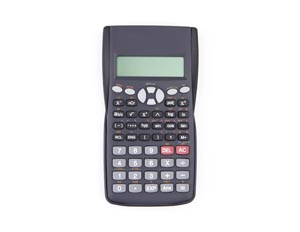StoreSeries • Module: Scientific Calculator UseModule: Scientific Calculator UseScientific Calculator Use

This module introduces the basic functions of a scientific calculator. The topics in the module include how to perform basic mathematical operations, such as addition, subtraction, multiplication, and division, as well as order of operations. The Scientific Calculator Use module also explains how to calculate percentages and square roots and how to enter numbers in scientific notations. In addition, this module describes how to use the trigonometric function of a scientific calculator.

This module takes 30 minutes to complete. At the end of this module, you must obtain an 80% or higher on the Test Your Knowledge for it to be marked as complete. You have unlimited attempts and the highest attempt is recorded.

 \$10.00